# RS Aggarwal Solutions for Class 6 Chapter 1 Number System Exercise 1D

RS Aggarwal Solutions for Class 6 Chapter 1 Number System Exercise 1D in PDF format is available here. Students who solve problems of RS Aggarwal textbook using the solutions will understand the chapter 1 Number System Exercise 1D thoroughly. The solutions mainly help students to gain a better hold on concepts that are covered under each exercise. Exercise 1D explains concepts like estimation and rounding off the numbers to the nearest tens, hundreds and thousands.

## Download PDF of RS Aggarwal Solutions for Class 6 Chapter 1 Number System Exercise 1D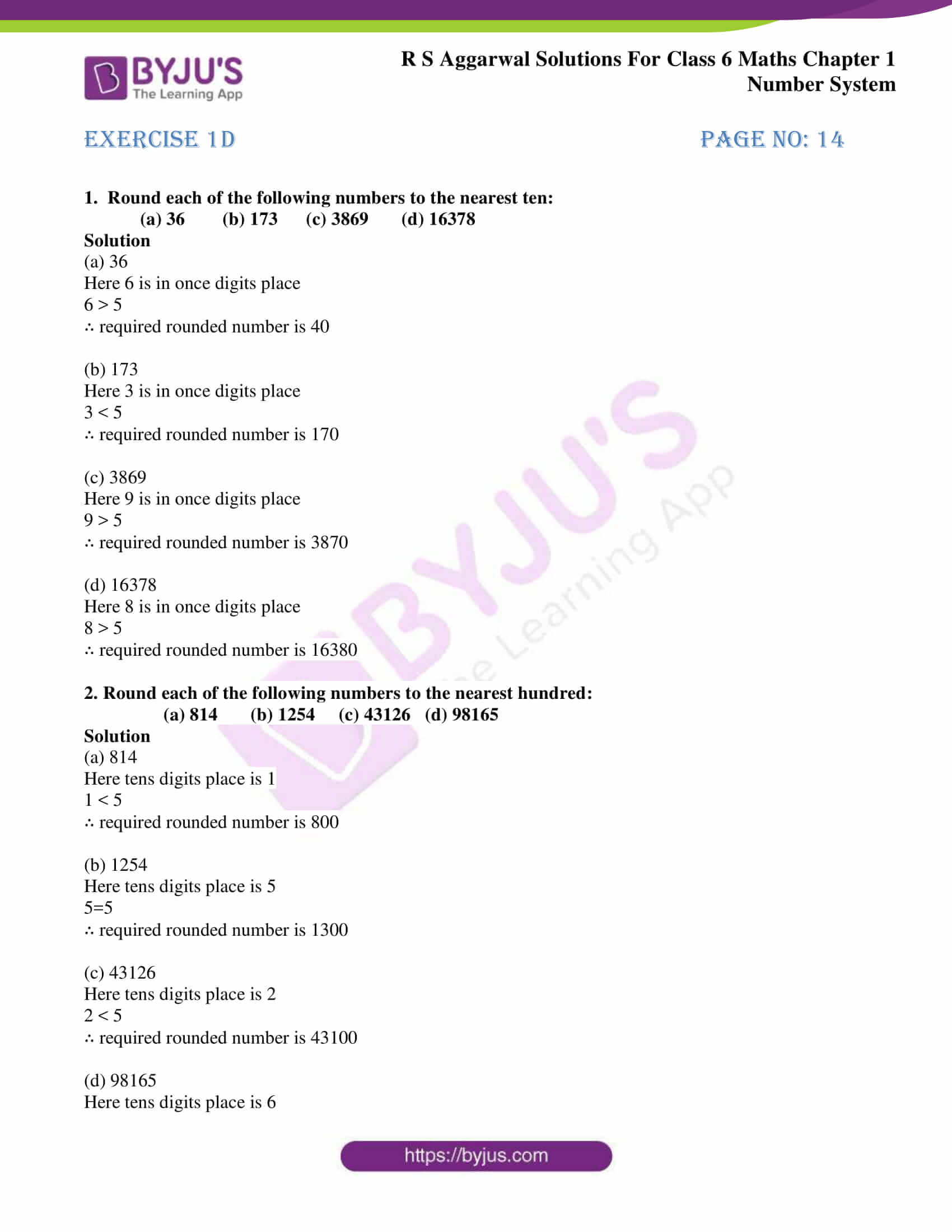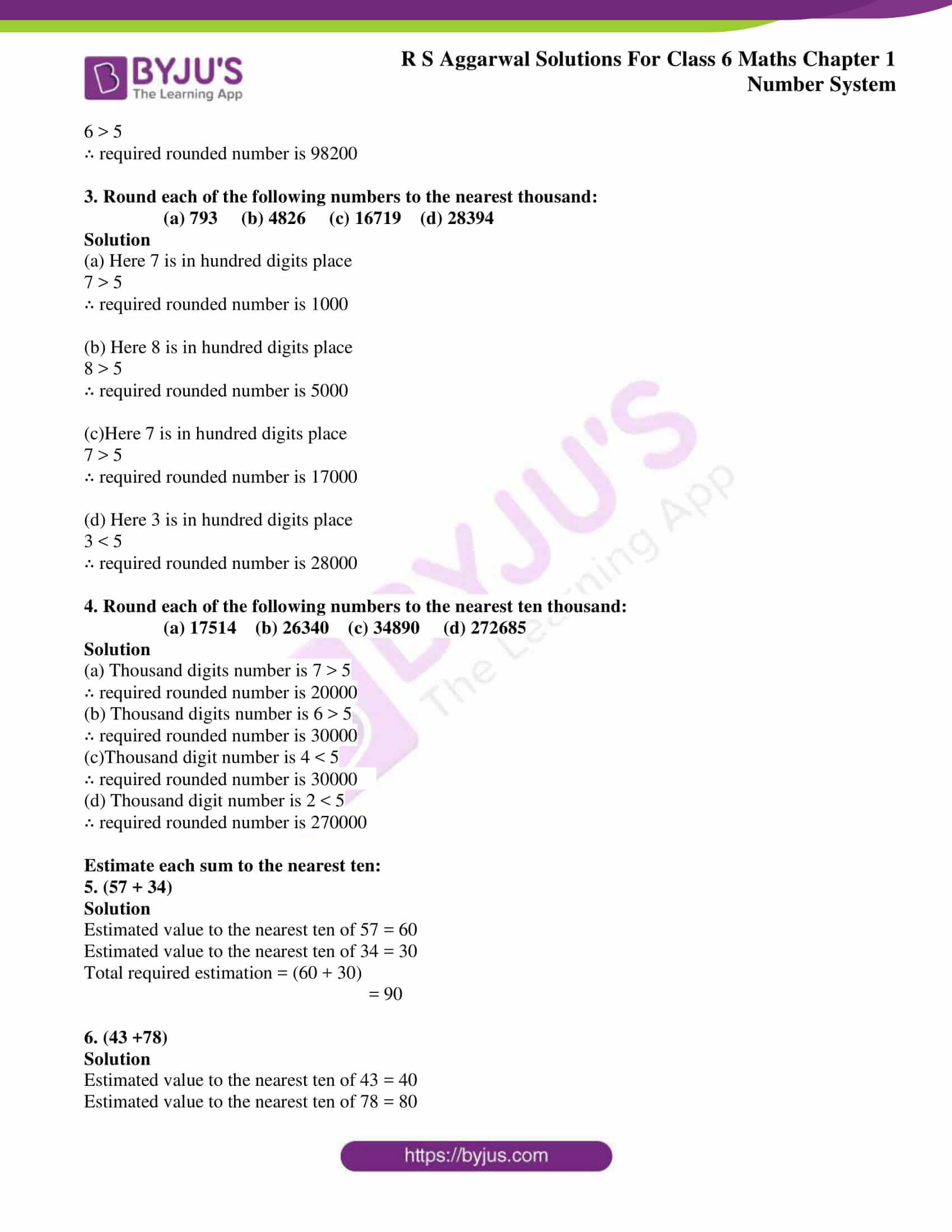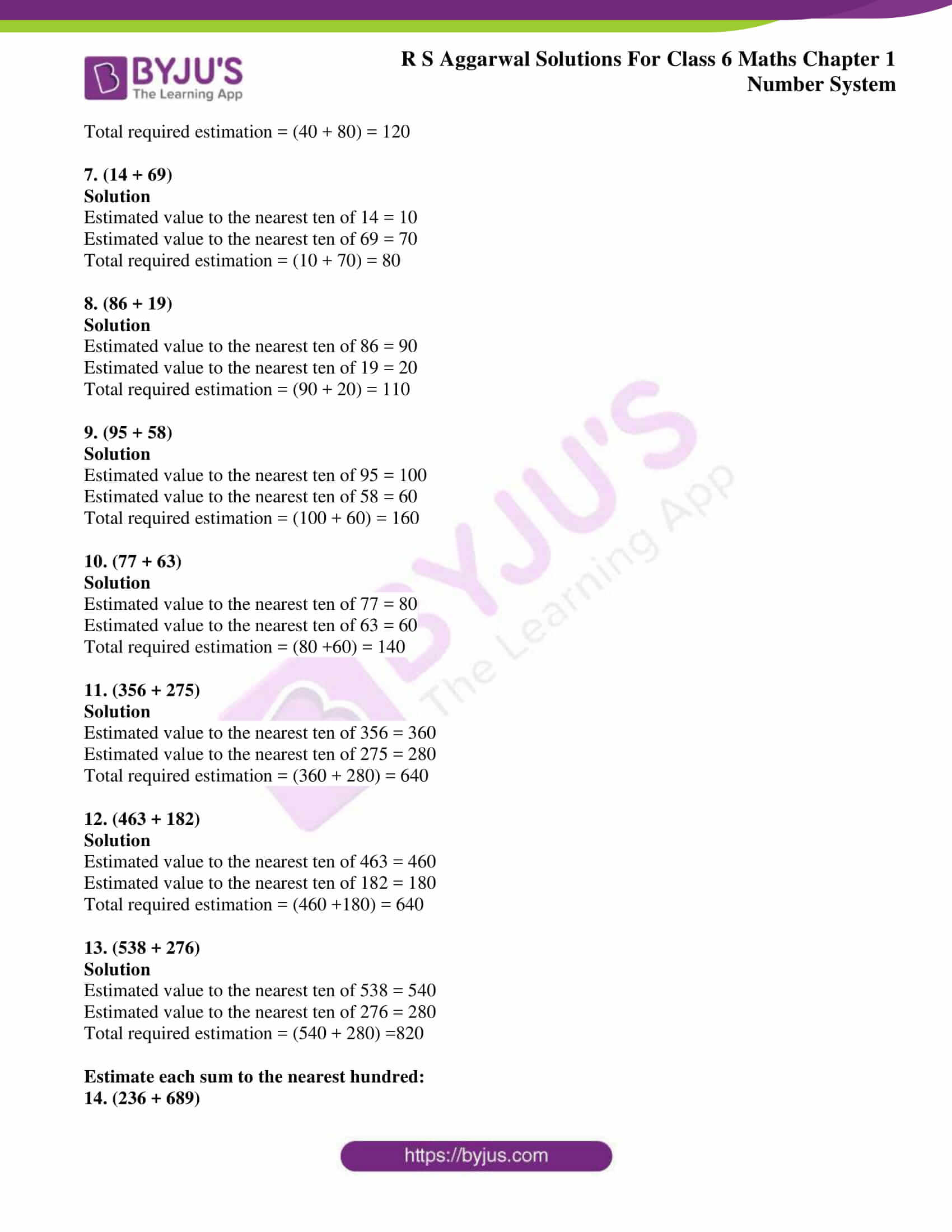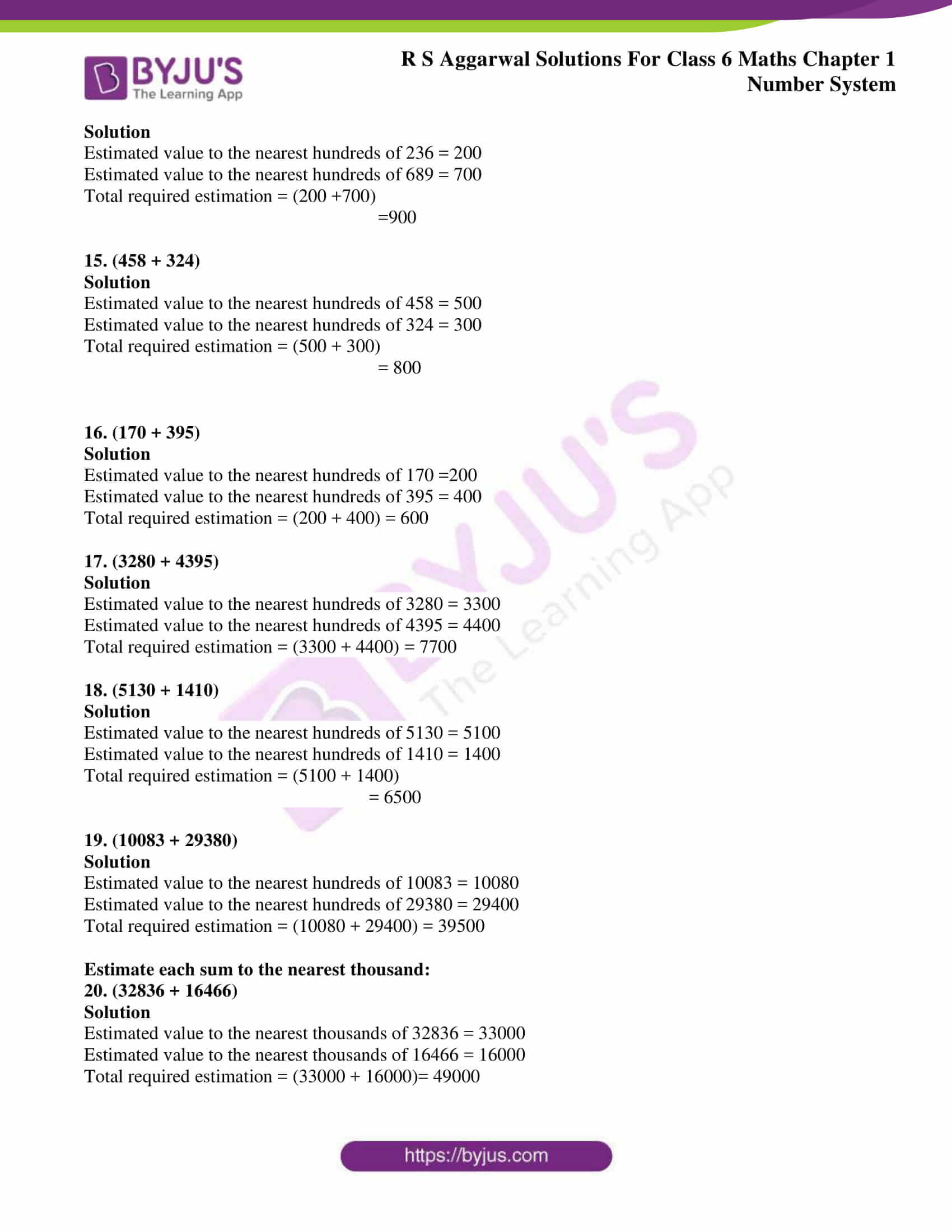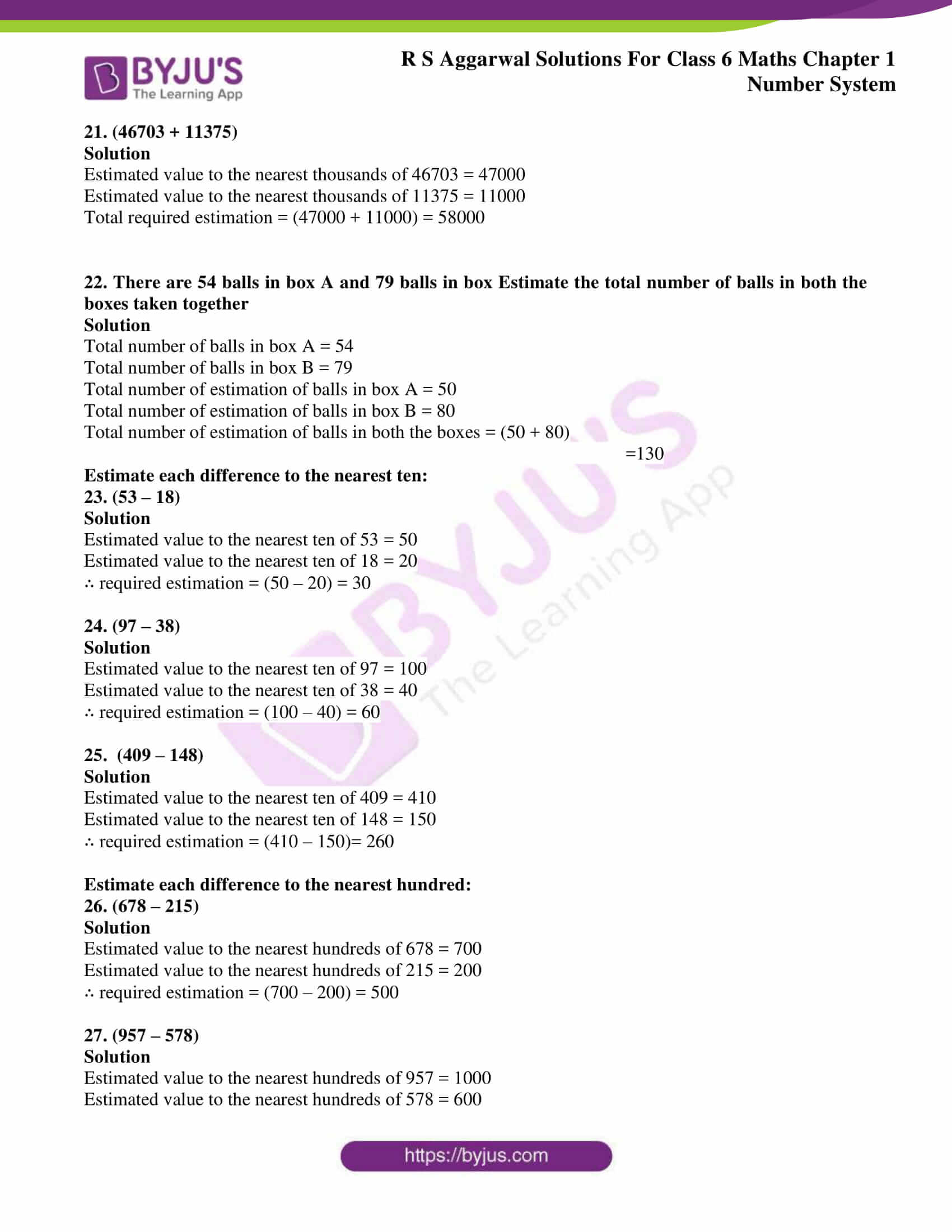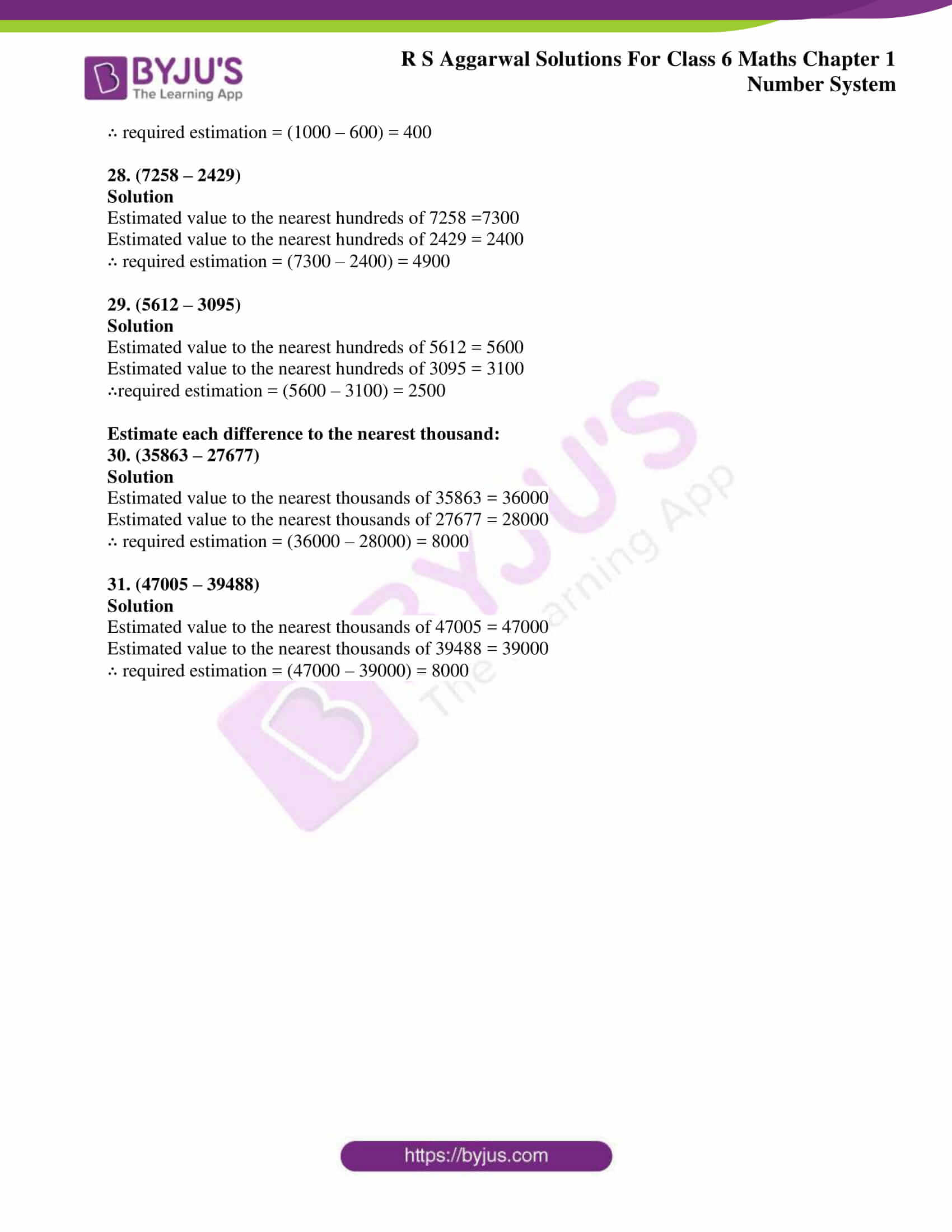### Access answers to Maths RS Aggarwal Solutions for Class 6 Chapter 1 Number System Exercise 1D

1. Round each of the following numbers to the nearest ten:

(a) 36 (b) 173 (c) 3869 (d) 16378

Solution

(a) 36

Here 6 is in once digits place

6 > 5

∴ required rounded number is 40

(b) 173

Here 3 is in once digits place

3 < 5

∴ required rounded number is 170

(c) 3869

Here 9 is in once digits place

9 > 5

∴ required rounded number is 3870

(d) 16378

Here 8 is in once digits place

8 > 5

∴ required rounded number is 16380

2. Round each of the following numbers to the nearest hundred:

(a) 814 (b) 1254 (c) 43126 (d) 98165

Solution

(a) 814

Here tens digits place is 1

1 < 5

∴ required rounded number is 800

(b) 1254

Here tens digits place is 5

5=5

∴ required rounded number is 1300

(c) 43126

Here tens digits place is 2

2 < 5

∴ required rounded number is 43100

(d) 98165

Here tens digits place is 6

6 > 5

∴ required rounded number is 98200

3. Round each of the following numbers to the nearest thousand:

(a) 793 (b) 4826 (c) 16719 (d) 28394

Solution

(a) Here 7 is in hundred digits place

7 > 5

∴ required rounded number is 1000

(b) Here 8 is in hundred digits place

8 > 5

∴ required rounded number is 5000

(c)Here 7 is in hundred digits place

7 > 5

∴ required rounded number is 17000

(d) Here 3 is in hundred digits place

3 < 5

∴ required rounded number is 28000

4. Round each of the following numbers to the nearest ten thousand:

(a) 17514 (b) 26340 (c) 34890 (d) 272685

Solution

(a) Thousand digits number is 7 > 5

∴ required rounded number is 20000

(b) Thousand digits number is 6 > 5

∴ required rounded number is 30000

(c)Thousand digit number is 4 < 5

∴ required rounded number is 30000

(d) Thousand digit number is 2 < 5

∴ required rounded number is 270000

Estimate each sum to the nearest ten:

5. (57 + 34)

Solution

Estimated value to the nearest ten of 57 = 60

Estimated value to the nearest ten of 34 = 30

Total required estimation = (60 + 30)

= 90

6. (43 +78)

Solution

Estimated value to the nearest ten of 43 = 40

Estimated value to the nearest ten of 78 = 80

Total required estimation = (40 + 80) = 120

7. (14 + 69)

Solution

Estimated value to the nearest ten of 14 = 10

Estimated value to the nearest ten of 69 = 70

Total required estimation = (10 + 70) = 80

8. (86 + 19)

Solution

Estimated value to the nearest ten of 86 = 90

Estimated value to the nearest ten of 19 = 20

Total required estimation = (90 + 20) = 110

9. (95 + 58)

Solution

Estimated value to the nearest ten of 95 = 100

Estimated value to the nearest ten of 58 = 60

Total required estimation = (100 + 60) = 160

10. (77 + 63)

Solution

Estimated value to the nearest ten of 77 = 80

Estimated value to the nearest ten of 63 = 60

Total required estimation = (80 +60) = 140

11. (356 + 275)

Solution

Estimated value to the nearest ten of 356 = 360

Estimated value to the nearest ten of 275 = 280

Total required estimation = (360 + 280) = 640

12. (463 + 182)

Solution

Estimated value to the nearest ten of 463 = 460

Estimated value to the nearest ten of 182 = 180

Total required estimation = (460 +180) = 640

13. (538 + 276)

Solution

Estimated value to the nearest ten of 538 = 540

Estimated value to the nearest ten of 276 = 280

Total required estimation = (540 + 280) =820

Estimate each sum to the nearest hundred:

14. (236 + 689)

Solution

Estimated value to the nearest hundreds of 236 = 200

Estimated value to the nearest hundreds of 689 = 700

Total required estimation = (200 +700)

=900

15. (458 + 324)

Solution

Estimated value to the nearest hundreds of 458 = 500

Estimated value to the nearest hundreds of 324 = 300

Total required estimation = (500 + 300)

= 800

16. (170 + 395)

Solution

Estimated value to the nearest hundreds of 170 =200

Estimated value to the nearest hundreds of 395 = 400

Total required estimation = (200 + 400) = 600

17. (3280 + 4395)

Solution

Estimated value to the nearest hundreds of 3280 = 3300

Estimated value to the nearest hundreds of 4395 = 4400

Total required estimation = (3300 + 4400) = 7700

18. (5130 + 1410)

Solution

Estimated value to the nearest hundreds of 5130 = 5100

Estimated value to the nearest hundreds of 1410 = 1400

Total required estimation = (5100 + 1400)

= 6500

19. (10083 + 29380)

Solution

Estimated value to the nearest hundreds of 10083 = 10080

Estimated value to the nearest hundreds of 29380 = 29400

Total required estimation = (10080 + 29400) = 39500

Estimate each sum to the nearest thousand:

20. (32836 + 16466)

Solution

Estimated value to the nearest thousands of 32836 = 33000

Estimated value to the nearest thousands of 16466 = 16000

Total required estimation = (33000 + 16000)= 49000

21. (46703 + 11375)

Solution

Estimated value to the nearest thousands of 46703 = 47000

Estimated value to the nearest thousands of 11375 = 11000

Total required estimation = (47000 + 11000) = 58000

22. There are 54 balls in box A and 79 balls in box Estimate the total number of balls in both the boxes taken together

Solution

Total number of balls in box A = 54

Total number of balls in box B = 79

Total number of estimation of balls in box A = 50

Total number of estimation of balls in box B = 80

Total number of estimation of balls in both the boxes = (50 + 80)

=130

Estimate each difference to the nearest ten:

23. (53 – 18)

Solution

Estimated value to the nearest ten of 53 = 50

Estimated value to the nearest ten of 18 = 20

∴ required estimation = (50 – 20) = 30

24. (97 – 38)

Solution

Estimated value to the nearest ten of 97 = 100

Estimated value to the nearest ten of 38 = 40

∴ required estimation = (100 – 40) = 60

25. (409 – 148)

Solution

Estimated value to the nearest ten of 409 = 410

Estimated value to the nearest ten of 148 = 150

∴ required estimation = (410 – 150)= 260

Estimate each difference to the nearest hundred:

26. (678 – 215)

Solution

Estimated value to the nearest hundreds of 678 = 700

Estimated value to the nearest hundreds of 215 = 200

∴ required estimation = (700 – 200) = 500

27. (957 – 578)

Solution

Estimated value to the nearest hundreds of 957 = 1000

Estimated value to the nearest hundreds of 578 = 600

∴ required estimation = (1000 – 600) = 400

28. (7258 – 2429)

Solution

Estimated value to the nearest hundreds of 7258 =7300

Estimated value to the nearest hundreds of 2429 = 2400

∴ required estimation = (7300 – 2400) = 4900

29. (5612 – 3095)

Solution

Estimated value to the nearest hundreds of 5612 = 5600

Estimated value to the nearest hundreds of 3095 = 3100

∴required estimation = (5600 – 3100) = 2500

Estimate each difference to the nearest thousand:

30. (35863 – 27677)

Solution

Estimated value to the nearest thousands of 35863 = 36000

Estimated value to the nearest thousands of 27677 = 28000

∴ required estimation = (36000 – 28000) = 8000

31. (47005 – 39488)

Solution

Estimated value to the nearest thousands of 47005 = 47000

Estimated value to the nearest thousands of 39488 = 39000

∴ required estimation = (47000 – 39000) = 8000

## RS Aggarwal Solutions for Class 6 Maths Chapter 1 Number System Exercise 1D

Exercise 1D of RS Aggarwal Solutions for Class 6 Chapter 1 Number System is based on the topic Estimation and rounding off numbers to the nearest tens, hundreds and thousands. Students are suggested to follow RS Aggarwal solutions which helps in grasping the topics of Exercise 1D easily. Topics which are covered in other exercises are:

• International system of numeration
• Comparison of numbers
• Estimating the products
• Estimating the quotients
• Roman numerals

Students who wish to understand the chapter without any obstacles are advised to go through RS Aggarwal solutions and practice to boost their exam preparation. It helps students to expertise in the subject which is important to maintain a good academic score.